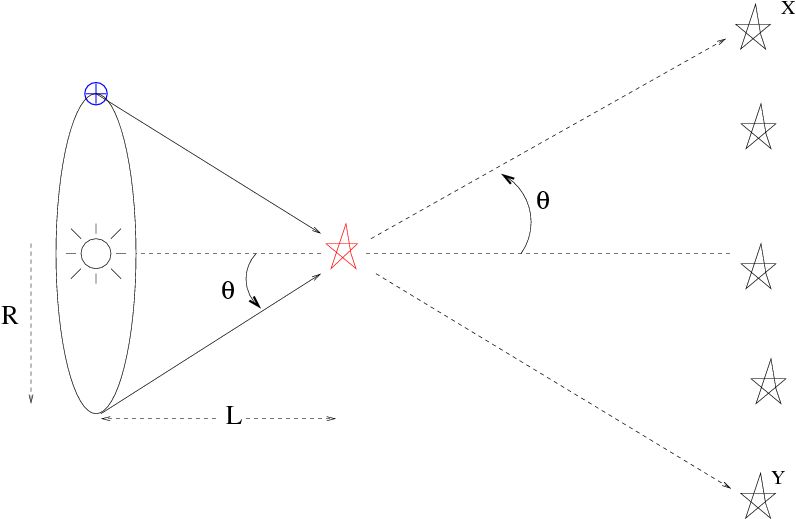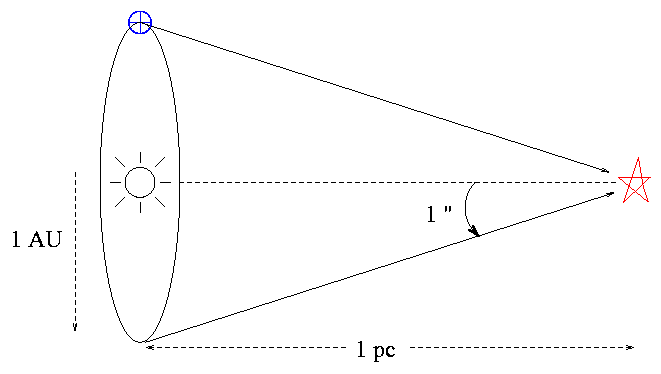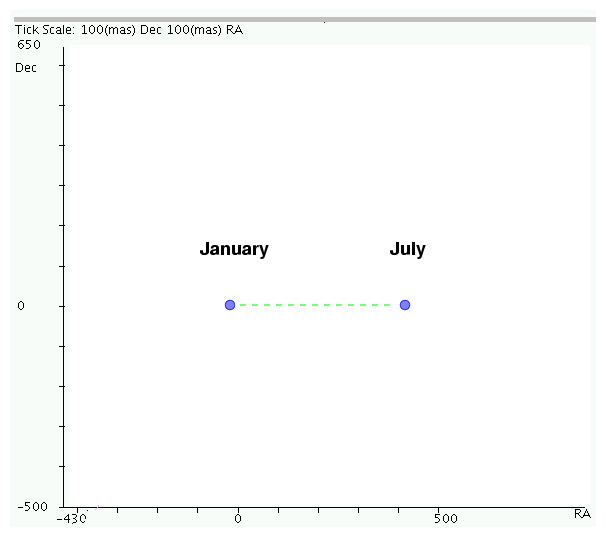Copyright © Michael Richmond. This work is licensed under a Creative Commons License.

# Measuring distances to stars via parallax

Remember measuring the distance to an asteroid by analyzing its apparent position in simultaneous images taken at two locations on Earth? That technique, called parallax, can also be used to measure the distances to some nearby stars ... if one modifies the observations a bit.

The simplest way to measure the distance to an object via parallax is to make simultaneous measurements from two locations on Earth. However, this method only works for objects which are relatively nearby:

```
Object        maximum possible parallax
(arcseconds)
-------------------------------------------------
Mars                 34
Jupiter               4
Neptune               0.6
Proxima Centauri      0.00007
-------------------------------------------------
```

The smallest angle one can measure from the ground with an optical telescope is about 0.003 arcseconds. It seems that simultaneous measurements from two locations will only work for bodies within our own solar system. We need to find some larger baseline to measure the parallax to other stars....

#### A longer baseline: the orbit of the Earth around the Sun

Astronomers need a VERY long baseline in order to produce a parallax angle which is large enough to detect via conventional imaging. Fortunately, there is a convenient baseline just waiting to be used -- if we are willing to discard the requirement that measurements be simultaneous (more on this later). This longer baseline is the radius of the Earth's orbit around the Sun:Note the convention used only in this situation: the quoted "heliocentric parallax angle" θ is always HALF the apparent angular shift.

So, if we measure a parallax half-angle θ to a star, we can calculate its distance very simply:

```
R
L   =   ---------
tan(θ)
```
where
```
6
R   =   149.6 x 10   km

=   1 Astronomical Unit (AU)

```

#### Small angles and peculiar units make life easy

Now, for small angles -- and the angles are always small for stars -- we can use the small angle approximations:

```
tan(θ) ~=  sin(θ)  ~=  θ
```
as long as we measure the angle in radians. Even if we don't use radians, it still remains true that cutting a small angle in half will also cut its tangent in half
```
θ           1
tan( --- )  ~=   --- tan( θ )
2           2
```
and, in general, there is a linear relationship between the tiny angle θ and its tangent, regardless of the units. Take a look for yourself:
```
angle        angle        tan(angle)
--------------------------------------------
1          0.017453        0.017455
0.5        0.008727        0.008727
0.1        0.0017453       0.0017453
0.01       0.00017453      0.00017453
--------------------------------------------
```

Okay, fine. Why am I belaboring this point? Because astronomers have chosen a set of units for parallax calculations which look strange, but turn out to simplify the actual work. The relationships between these units depend on the fact that there is a simple linear relationship between a tiny parallax angle, in ANY units, and the tangent of that angle.

angles are measured in arcseconds (")
Recall that 1 arcsecond = 1/3600 degree. Astronomers use arcseconds because parallax values for nearby stars are just a bit less than one arcsecond. You may also see angles quoted in mas, which stand for milli-arcseconds: 1 mas = 1/1000 arcsecond.

distances are measured in parsecs (pc)
A parsec is defined as the distance at which a star will have a heliocentric parallax half-angle of 1 arcsecond.```
Q: How many meters are in one parsec?

How many light years are in one parsec?
```

Given these units, and the linear relationship between a small angle and its tangent, we can calculate the distance to a star (in pc) very simply if we know its parallax half-angle in arcseconds:

```
1
distance (pc)  =  -----
θ
```

Give it a try: the first star to have its parallax measured was 61 Cygni. In 1838, Friedrich Bessel calculated its parallax half-angle to be θ = 0.314 arcsec.

```

Q:  What is the distance to 61 Cygni based on Bessel's measurement?

```

#### Some observational difficulties

It sounds pretty easy to measure the distance to another star -- just make measurements of its position six months apart. In practice, however, it is very difficult. The first successful measurement of stellar parallax came more than two hundred years after the invention of the telescope. What took astronomers so long?

• The parallax shifts are always small.

Really small. Smaller than the apparent size of stars as seen from the Earth's surface. Starlight is refracted by air as it passes through the Earth's atmosphere. It encounters layers with a range of temperatures and pressures, and, what's worse, all these layers are constantly in motion.

As a result of all this refraction, astronomers on the ground perceive stars to be little blurry spots. The typical size of a "seeing disk" is around 1 arcsecond. In order to measure the parallax of a star, we must determine its position -- and that of several reference stars in the same field -- to a very small fraction of this seeing disk. That's not an easy task.

• All stars in a field exhibit parallax

In practice, astronomers usually measure the shift of one star in an image relative to other stars in the same image; differential measurements can be made much more precisely than absolute ones. However, as the Earth moves from one side of the Sun to the other, we will see ALL the stars in the field shift, not only the star of interest.

The only hope is to pick out a set of reference stars which happen to be much farther away than the target star. Distant stars will shift by a much smaller angle -- perhaps small enough to be imperceptible. If we measure the position of the nearby target star relative to those distant ones, we might be able to detect its shift.

• Heliocentric parallax measurements are not simultaneous

We have to wait months for the Earth to move a significant distance in its orbit around the Sun. During that time, the Sun is moving through space at a decent clip: very roughly 12 km/s relative to nearby stars in the disk of the Milky Way. Other stars are moving, too, by similar speeds. The resulting proper motions can be signficant at the level of precision required for parallax measurements.

For example, when we look at the nearby star Vega, we might expect it to move in a simple line between two apparent positions:But its real motion shows the combination of parallax and proper motion. Consider these observations of Vega over a period of three years, made by the Hipparcos satellite.

#### The bottom line

The best large set of parallax measurements come from the Hipparcos satellite, which measured the position and brightness of relatively bright (brighter than tenth magnitude or so) stars over the entire sky during the period 1989-1993. A large team of scientists turned its millions of raw measurements into a consistent catalog of distances and luminosities.

The precision of the Hipparcos measurements of parallax is about 0.002 arcsecond. That means that we can rely on Hipparcos measurements to determine distances out to 100 parsecs or a bit further.

#### Coming soon: Gaia, a bigger, better astrometry mission

The European Space Agency is planning to launch in 2013 the Gaia satellite, which is very much like a big brother to Hipparcos. It will also measure the positions and distances to stars, but do a better job:

```
Hipparcos              Gaia
----------------------------------------------------------
Telescope diam (cm)       29                 ~ 100

Mag limit                 12                   20

# stars (approx)        100,000          1,000,000,000

best precision           0.001               0.000 010
(arcsec)

velocity measures        none               1-10 km/s  at V=16

----------------------------------------------------------
```

#### Homework

1. Way back in 1838, Bessel determined the parallax to 61 Cygni to be 0.314 arcseconds. How good was his measurement? You can check it against a more modern measurement by the Hipparcos satellite.
1. The star 61 Cygni appears in the Hipparcos catalog as star number 104214. Use the Hipparcos Intermediate Data Search Facility or the SIMBAD catalog search site to find the star's parallax, as measured by Hipparcos.
2. What is the percentage difference between the Hipparcos angle and Bessel's angle? (The abbreviation "mas" stands for "milli-arcseconds")
3. Use the Hipparcos parallax angle to calculate the distance to the star.
4. What is the percentage difference between the Hipparcos distance and Bessel's distance?
2. Computing the uncertainty in distance to a star based on its parallax can be a tricky thing. Consider the case of Joe and the star Rigel. Joe measures a parallax half-angle of θ = 0.004 arcsec to Rigel. He estimates the uncertainty in his measurement to be +/- 0.003 arcsec. In other words, the parallax could be as large as 0.007 arcsec, or as small as 0.001 arcsec.
1. What is the distance to Rigel, if Joe's measurement is exactly correct?
2. What is the distance to Rigel, if the error in Joe's measurement is +0.003 arcsec (i.e. if Joe's measurement is at the lower end of its estimated uncertainty)?
3. What is the distance to Rigel, if the error in Joe's measurement is -0.003 arcsec (i.e. if Joe's measurement is at the upper end of its estimated uncertainty)?
Bonus! What is the actual distance to Rigel? How well do we know it?Copyright © Michael Richmond. This work is licensed under a Creative Commons License.